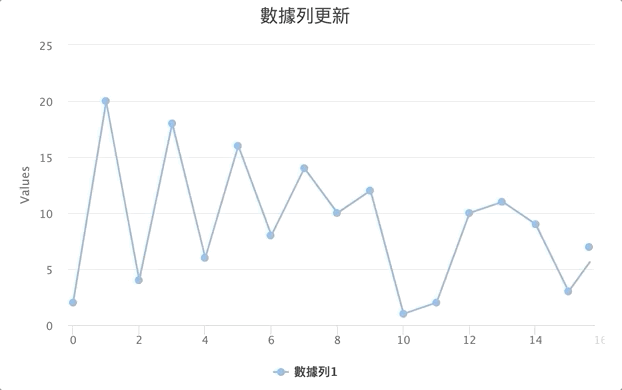# 資視就是力量 - Highcharts / 資料更新 Method

Oct 3, 2020 Max Lee

## 資料更新 Method

``````// 創建一個圖表，設定內容省略
var chart = Highcharts.chart(container, options);

// 圖表的更新 Method
chart.update();
// 數據列的更新 Method
chart.series.update();
chart.series.remove();
chart.series.setData();
chart.series.removePoint();
// 數據點的更新 Method
chart.series.data.update();
chart.series.data.remove();
``````

## 整體圖表更新 Method

#### # chart.update(options)

`options: Object`

``````var chart = Highcharts.chart(container, {
title: { text: "我的圖表" },
credits: { enabled: false },
series: [
{ name: "數據列1", data: [1,2,3] },
{ name: "數據列2", data: [4,5,6] }
]
});
// 只有第一組數據列會被更新，但第二組並不會消失
chart.update({
title: { text: "我的圖表(已更新)" },
series: [{ data: [10,20,30] }]
});
``````

`series: Object`

``````chart.addSeries({
name: "新數據列",
dataLabels: { enabled: true },
data: [[10,20], [30,40], [50,60]]
});
``````

## 數據列更新 Method

#### # series.update(options)

`options: Object`

``````var chart = Highcharts.chart(container, {
series: [
{ name: "數據列1", data: [1,2,3] },
{ name: "數據列2", data: [4,5,6] }
]
});
// 第二組數據列將被更新
chart.series.update({
name: "數據列2(被更新)",
color: "red",
data: [40, 50, 60]
});
``````

#### # series.remove()

``````chart.series.remove();
``````

#### # series.setData(data)

`data: Array`

``````var chart = Highcharts.chart(container, {
series: [{ data: [1,2,3] }]
});
// 數據列的數據將會改變
chart.series.setData([4,5,6]);
``````

`point: Number|Array|Object`

``````chart.series.addPoint({x: 10, y: 10});
``````

#### # series.removePoint(index)

`index: Number`

``````var chart = Highcharts.chart(container, {
series: [{ data: [6,7,8,9,10] }]
});
// 第三個數據點將會被移除
chart.series.removePoint(2);
``````

## 數據點更新 Method

#### # point.update(options)

`options: Number|Array|Object`

``````var chart = Highcharts.chart(container, {
series: [{ data: [10, 11, 12, 13, 14] }]
});
// 第四個數據點將被更新
chart.series.data.update({ y: 20, color: "red" });
``````

#### # point.remove()

``````chart.series.data.remove();
``````

## 指定選取

#### # series.id / series.data.id

`型別: String` `預設: undefined`

``````var myChart = Highcharts.chart(container, {
series: [{
id: "mySeries",
data: [1, 2, {y: 3, id: "myPoint" }]
}]
});

let series = myChart.get("mySeries");
let point = myChart.get("myPoint");
``````- 此篇文章為「iT邦幫忙鐵人賽」參賽文章，同步發表於 iT邦幫忙 -